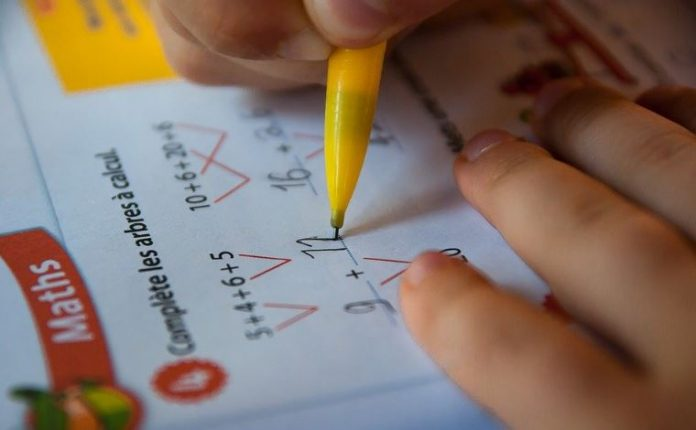In the Class 9 NCERT Maths book, every concept of each chapter is described concisely and has practical relevance of all the concepts as well. Students need to understand the fundamentals first and then move ahead with the practising of the exercises. However, if you find difficulty in solving the questions from the NCERT exercises, then you should definitely refer to the Vedantu Maths NCERT solutions Class 9. Prepared by the experts in the industry, these solutions can help you gain a better understanding of all the concepts chapter-wise.

For your convenience, an overview of the NCERT Class 9 Maths textbook and the number of exercises per chapter is provided in the following.

### How referring to NCERT solutions for Class 9 will help in understanding the fundamentals of Mathematics?

Mathematics in Class 9 could be a bit difficult for students to understand as you are moving forward to the first board exam in your life. Hence there will be a few new chapters and topics to understand. You might face a bit of difficulty to understand the new concepts. But there’s nothing to worry about! You will be able to understand it all if you put in a little effort.

Understanding the basic concepts will help you to score well in the exams. But not only understanding the fundamental concepts but also you will have to solve different problems from your NCERT Maths book and analyse your own solved problems. But how to do it? There are several solutions to the NCERT textbook offered by various e-learning portals or ed-tech platforms such as Vedantu.

These solutions are very thorough and excellently curated by the highly qualified experts in the academic field. With the help of these solutions, you will be able to learn how to solve a problem in an easy way and in comparatively less time. Besides, you will be aware of the question patterns and the chapter-wise marks weightage as well. So, you can come up with a brilliant exam strategy. So that you can complete your textbook ahead of your exam and get enough time to practise the exercises from the book. That will really help you gain confidence and make you prepared for the exam as well.

Class 9 Maths chapter wise overview:

> Chapter 1 – Number System

 S.No Sub-topics 1.1 Introduction 1.2 Irrational Numbers 1.3 Real Numbers and Their Decimal Expansions 1.4 Representing Real Numbers on the Number Line 1.5 Operations on Real Numbers 1.6 Laws of Exponents for Real Numbers 1.7 Summary

> Chapter 2 – Polynomial

 S.No Sub-topics 2.1 Introduction 2.2 Polynomials in One Variable 2.3 Zeros of a Polynomials 2.4 Remainder Theorem 2.5 Factorisation of Polynomials 2.6 Algebraic Identities 2.7 Summary

> Chapter 3 – Coordinate Geometry

 S.No Sub-topics 3.1 Introduction 3.2 Cartesian System 3.3 Plotting a Point in the Plane if its Coordinates are given 3.4 Summary

> Chapter 4 – Linear Equations in Two Variables

 S.No Sub-topics 4.1 Introduction 4.2 Linear Equations 4.3 Solutions of a Linear Equation 4.4 Graph of a Linear Equation in Two Variables 4.5 Equations of Lines Parallel to x-axis and y-axis 4.6 Summary

> Chapter 5 – Introduction to Euclid’s Geometry

 S.No Sub-topics 5.1 Introduction 5.2 Euclid’s Definitions, Axioms and Postulates 5.3 Equivalent versions of Euclid’s Fifth Postulate 5.4 Summary

> Chapter 6 – Lines and Angles

 S.No Sub-topics 6.1 Introduction 6.2 Basic Terms and Definitions 6.3 Intersecting Lines and Non-intersecting Lines 6.4 Pairs of Angles 6.5 Parallel Lines and a Transversal 6.6 Lines Parallel to the same Line 6.7 Angle Sum Property of a Triangle 6.8 Summary

> Chapter 7 – Triangles

 S.No Sub-topics 7.1 Introduction 7.2 Congruence of Triangles 7.3 Criteria for Congruence of Triangles 7.4 Some Properties of a Triangle 7.5 Some More Criteria for Congruence of Triangles 7.6 Inequalities in a Triangles 7.7 Summary

 S.No Sub-topics 8.1 Introduction 8.2 Angle Sum Property of a Quadrilateral 8.3 Types of Quadrilaterals 8.4 Properties of a Parallelogram 8.5 Another Condition for a Quadrilateral to be a Parallelogram 8.6 The Mid-point Theorem 8.7 Summary

>Chapter 9 – Areas of Parallelograms

 S.No Sub-topics 9.1 Introduction 9.2 Figures on the same Base and Between the Same Parallels 9.3 Parallelograms on the same Base and between the same Parallels 9.4 Triangles on the same Base and between the same Parallels 9.5 Summary

> Chapter 10 – Circles

 S.No Sub-topics 10.1 Introduction 10.2 Circles and its Related Terms: A Review 10.3 Angle Subtended by a Chord at a Point 10.4 Perpendicular from the Centre to a Chord 10.5 Circle through Three Points 10.6 Equal Chords and their Distances from the Centre 10.7 Angle Subtended by an Arc of a Circle 10.8 Cyclic Quadrilaterals 10.9 Summary

> Chapter 11 – Constructions

 S.No Sub-topics 11.1 Introduction 11.2 Basic Constructions 11.3 Some Constructions of Triangles 11.4 Summary

> Chapter 12 – Heron’s Formula

 S.No Sub-topics 12.1 Introduction 12.2 Area of a Triangle – by Heron’s Formula 12.3 Application of Heron’s Formula in finding 12.4 Summary

> Chapter 13 – Surface Areas and Volumes

 S.No Sub-topics 13.1 Introduction 13.2 Surface Area of a Cuboid and a Cube 13.3 Surface Area of a Right Circular Cylinder 13.4 Surface Area of a Right Circular Cone 13.5 Surface Area of a Sphere 13.6 Volume of a Cuboid 13.7 Volume of a Cylinder 13.8 Volume of a Right Circular Cone 13.9 Volume of a Sphere 13.10 Summary

> Chapter 14 – Statistics

 S.No Sub-topics 14.1 Introduction 14.2 Collection of Data 14.3 Graphical Representation of Data 14.4 Measures of Central Tendency 14.5 Summary

> Chapter 15 – Probability

 S.No Sub-topics 15.1 Introduction 15.2 Probability – an Experimental Approach 15.3 SummaryWith over 12 years of experience, she is a proficient content writer and editor specializing in a diverse range of subjects, including technology news, country news, arts, science, travel, and automobiles.